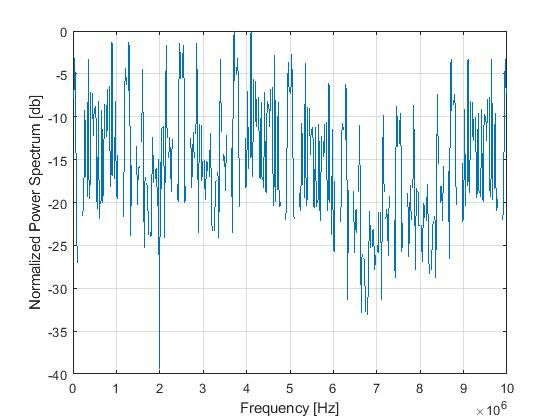## 1024 FFT for SPT simulation issues with model base design toolbox

cancel
Showing results for
Did you mean:
SOLVED

## 1024 FFT for SPT simulation issues with model base design toolbox

419 Views
Contributor I

I tried to simulate 1024 FFT using model base design toolbox.

The twiddle factor was generated by following code

NFFT =1024

for mm = 0:1:(NFFT-1)
theta = (-2*pi*mm*1/NFFT);
twiddle(mm+1) = cos(theta) + (1i*(sin(theta)));
end
twiddle = twiddle*0.99997*2^15;

The window function was generate as following

win = chebwin(NFFT,100);
win = round(win*0.99998*2^15).';

A cos signal with 10* time of  frequency resolution (delta_f) was used as test signal;

NFFT =1024;
fs = 20.e6;
delt_f = fs/NFFT;
f1 = 10* delt_f;

S = cos(2*pi*f1*[0:NFFT-1]/fs + (rand * 2 *pi)) * (0.25);

S = round(S * 2^11);
S = S .* 2^2;

Then it was passed to spt functions to simulate 1024 FFT results by following

win_out = win_mex(complex(win),complex(S),'MULTIPLE_COEFF',0);

However, it could not get correct peak at Stage_4 output. The results was followingIs anything I forgot or missed ?

Tags (3)
1 Solution
187 ViewsNXP Employee

Hello morrisli‌,

I attached a m script that has as starting point your code. The main problem was with twiddle factors. When you use quadrature extension, the first has to be cos(2*pi*1/NFFT)-sin(2*pi*1/NFFT), and you need only one eighth of them. Also you can apply the window in the first round of a radix 4 instruction, like this:

Stage_0 = rdx4_mex(complex(win), complex(S), 'WIN_ENABLED', 'MULTIPLE_COEFF', 0, 'QUAD_EXT', 'NO_COMBINED_FFT', 0);

Kind regards,

Iulian Bulancea

188 ViewsNXP Employee

Hello morrisli‌,

I attached a m script that has as starting point your code. The main problem was with twiddle factors. When you use quadrature extension, the first has to be cos(2*pi*1/NFFT)-sin(2*pi*1/NFFT), and you need only one eighth of them. Also you can apply the window in the first round of a radix 4 instruction, like this:

Stage_0 = rdx4_mex(complex(win), complex(S), 'WIN_ENABLED', 'MULTIPLE_COEFF', 0, 'QUAD_EXT', 'NO_COMBINED_FFT', 0);

Kind regards,

Iulian Bulancea